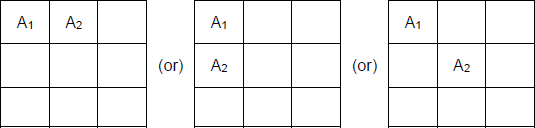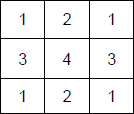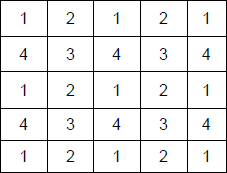# You are given an n×n square matrix to be ﬁlled with numerals | CAT DILR questions – Logical Reasoning

### Since 2015, the CAT DILR section has become increasingly difficult. In order to tackle the tougher CAT Level DILR questions for the CAT Exam, it is important to understand the basics of CAT. Data Interpretation sets and Logical reasoning puzzles were distinct in DILR. Data Interpretation and Logical Reasoning used to be about computation and understanding charts, graphs, and tables. Within that one hour, you get 5 high-quality puzzles, with some of them being quite challenging. You will solve 30 actual CAT puzzles in CAT 2018 and CAT 2019. The purpose of this page is to help you prepare for that. Take a crack at those puzzles!To obtain a great CAT score, make use of MBAP Free Study material with detailed solutions and video explanations. Check out MBAP free Mock test to take these questions in a test format for free.

You may also find remaining question solution of CAT 2018, slot 1 by searching the question in the search bar.

## CAT 2018 - Slot 1 - Set 3 - You are given an n×n square matrix to be ﬁlled with numerals

### Explanation:Given that n × n square matrix to be filled with numerals so that no two adjacent cells have the same numeral. Also, two cells are called adjacent if they touch each other horizontally, vertically or diagonally. As per the given definition, in the following matrix, the following are the cases of adjacent cells.Q. 1: What is the minimum number of different numerals needed to ?ll a 3×3 square matrix?

As per the information, we’ve the following diagram for a 3 x 3 matrix to have minimum number of numerals.So, we require 4 elements to have all different numerals. Ans : 4

##### Q. 2: What is the minimum number of different numerals needed to ?ll a 5×5 square matrix?

As per the information, we’ve the following diagram for a 5 x 5 matrix to have minimum number of numerals.So, we require 4 elements to have all different numerals. Ans : 4

Q. 3: Suppose you are allowed to make one mistake, that is, one pair of adjacent cells can have the same numeral. What is the minimum number of different numerals required to ?ll a 5×5 matrix?
1. 16
2. 4
3. 25
4. 9

Even if one mistake is allowed, then also there won’t be any change in the solution given above. Ans : 4

Q. 4: Suppose that all the cells adjacent to any particular cell must have different numerals. What is the minimum number of different numerals needed to fill a 5×5 square matrix?
1. 9
2. 16
3. 4
4. 25

Given that all the cells adjacent to any particular cell must have different numerals, which is satisfied only

when there are at least 9 numerals. Ans : 9

### Past Year Question Paper & SolutionsCounselling Session
By IIM Mentor

#### Free Material Area